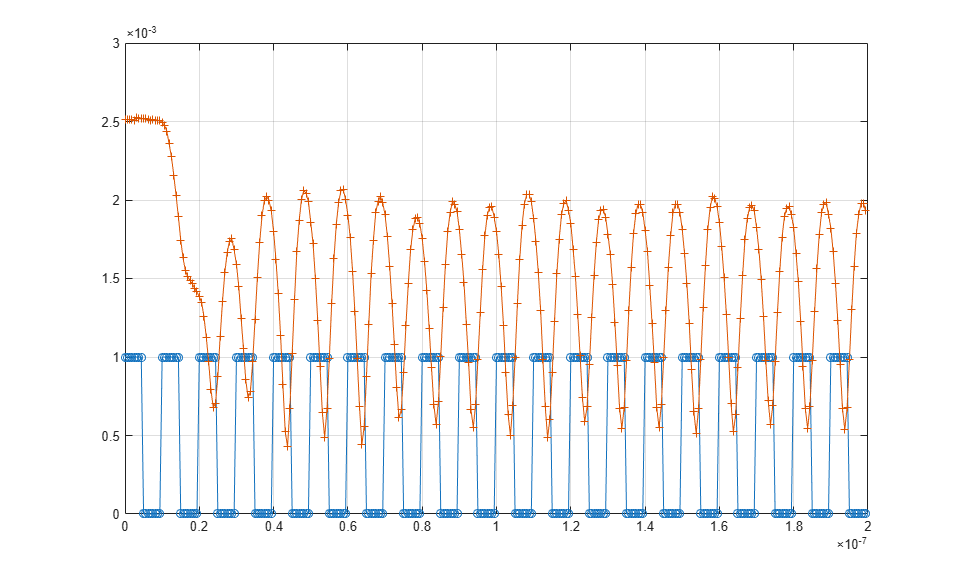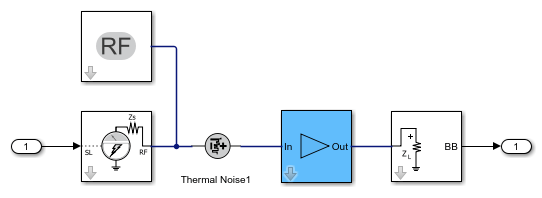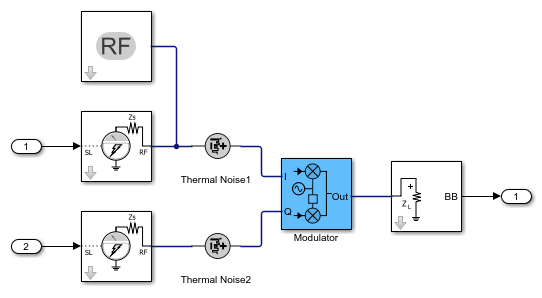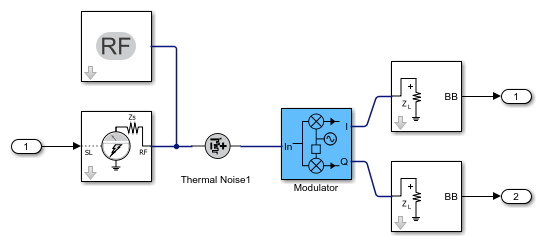rfsystem

Perform circuit envelope simulation of RF system designed using rfbudget

Description

Use the rfsystem System object™ to perform circuit envelope simulation of an RF system designed using an rfbudget object. You can use the rfsystem object to generate an RF Blockset™ model. This object supports vector inputs and has no frame-size limits.

To perform circuit envelope simulation of an RF system:

1. Create the rfsystem object and set its properties.

2. Call the object with arguments, as if it were a function.

Creation

Description

example

rfs = rfsystem(rfb) creates a System object from the RF system rfb. Use the System object, rfs, to perform circuit envelope simulation.

The System object generates an untitled RF Blockset model of the RF system. Use Object Functions to open, save, close, or hide the RF Blockset model.

example

rfs = rfsystem(rfb,Name,Value) sets Properties using name-value arguments. For example, rfsystem(rfb,'ModelName','rfmodel') sets the name of the RF Blockset model to rfmodel.

Properties

expand all

Unless otherwise indicated, properties are nontunable, which means you cannot change their values after calling the object. Objects lock when you call them, and the release function unlocks them.

If a property is tunable, you can change its value at any time.

Name of the RF Blockset model, specified as a string scalar or character vector.

Example: 'ModelName','RFModel'

Input frequency applied to each input of the RF system, specified as a non-negative scalar in Hz. This input frequency is derived from the RF system designed using an rfbudget object. Your RF system will have two inputs, I and Q, at DC if the rfb.InputFrequency is set to 0.

Note

You can set InputFrequency to be a vector if you want to investigate the intermodulation of nearby signals going through the same architecture after construction of the rfsystem system object.

Output frequency to be computed at the output of the RF system, specified as a non-negative in Hz. This output frequency is derived from the RF system designed using an rfbudget object. Your RF system will have two outputs, I and Q, at DC if the value of the rfb.OutputFrequency is 0.

Time step for circuit envelope simulation, specified as a positive scalar. This property sets the step size between simulations.

Usage

Description

example

out = rfs(in) creates an RF Blockset circuit envelope simulation output out using input signal values in. Pass in as an input argument to an automatically-generated RF Blockset model.

You can design four architectures, RF to RF, DC to RF, RF to DC, and DC to DC, using the rfsystem object. For more information, see Design RF-RF, IQ-RF, RF-IQ, and IQ-IQ Architectures.

Note

Passing multiple input vectors and concatenating the output vectors is equivalent to performing one long simulation with a vertically-concatenated input.

Input Arguments

expand all

Input time-domain signal, specified as column vectors. Specify in as an array of column vectors when there are N 'InputFrequency' values.

Output Arguments

expand all

Output time-domain signal, returned as column vectors. out is returned as an array of column vectors when there are N 'OutputFrequency' values to be computed.

Object Functions

To use an object function, specify the System object™ as the first input argument. For example, to release system resources of a System object named obj, use this syntax:

release(obj)

expand all

 open_system Open RF Blockset model created using rfsystem save_system Save RF Blockset model created using rfsystem close_system Close RF Blockset model window created using rfsystem hide_system Hide RF Blockset model window created using rfsystem

expand all

 step Run System object algorithm release Release resources and allow changes to System object property values and input characteristics reset Reset internal states of System object

Examples

collapse all

Design an RF receiver to perform circuit envelope simulation.

Create fifth- and seventh-order bandpass RF filters.

f1 = rffilter('ResponseType','Bandpass','FilterOrder',5, ...
'PassbandFrequency',[4.85 5.15]*1e9);
f2 = rffilter('ResponseType','Bandpass','FilterOrder',7, ...
'PassbandFrequency',[10 130]*1e6);

Create two amplifier objects with 3 dB and 5 dB gain, respectively.

a1 = amplifier('Gain',3,'NF',1.53,'OIP3',35);
a2 = amplifier('Gain',5,'NF',8,'OIP3',37);

Create a modulator with a local frequency of 4.93 GHz.

d = modulator('Gain',0,'NF',4,'OIP3',50,'LO',4.93e9, ...
'ConverterType','Down');

Design an RF receiver with the budget elements at an input frequency of 5 GHz, an available input power of -30 dBm, and a bandwidth of 200 MHz.

rfb = rfbudget([f1 a1 d f2 a2],5e9,-30,200e6);

Create an RF system for the RF receiver using the rfbudget object.

rfs = rfsystem(rfb);

Specify input time-domain signal for the RF system.

in = [1e-3*ones(8,1); zeros(8,1)] .* ones(1,10);
in = in(:);

Calculate the output time-domain signal of the RF system.

out = rfs(in);
out = [out; rfs(in)];

Specify the sample time of the RF system.

t = rfs.SampleTime*(0:length(out)-1);

Plot the simulated output.

plot(t,[in; in],'-o',t,abs(out),'-+')
grid onRelease system resources and turn off fast restart.

release(rfs)

Open an RF Blockset model of the designed RF system using the open_system object function.

open_system(rfs)Design four different chain architectures using an RF System object.

Create an input column vector.

in = (1:8)';

Design RF-RF Architecture

Create an rfbudget object using an amplifier object.

a = amplifier;

Calculate the RF budget of the amplifier at an input frequency of 5 GHz, an available input power of –30 dBm, and a bandwidth of 10 KHz.

rfb = rfbudget(a,5e9,-30,10e3);

Create an RF system using the rfbudget object.

rfs = rfsystem(rfb);

Create an RF-RF architecture using the input column vector.

out0 = rfs(in);

Release system resources and turn off fast restart.

release(rfs)

Open an RF Blockset model of the RF system.

open_system(rfs)Design IQ-RF Architecture

Use a modulator object with an up converter to create an rfbudget object.

u = modulator('ConverterType','Up','LO',1e9);

Calculate the RF budget of the modulator at an input frequency of 0 GHz, an available input power of –30 dBm, and a bandwidth of 10 KHz.

rfb2 = rfbudget(u,0,-30,10e3);

Create an RF system using the rfbudget object.

rfs2 = rfsystem(rfb2);

Create an IQ-RF architecture using the input column vector.

inI = in;
inQ = in;
out = rfs2(inI,inQ);

Release system resources and turn off fast restart.

release(rfs2)

Open an RF Blockset model of the RF system.

open_system(rfs2)Design RF-IQ Architecture

Use a modulator object with a down converter to create an rfbudget object.

d = modulator('ConverterType','Down','LO',1e9);

Calculate the RF budget of the modulator at an input frequency of 1 GHz, an available input power of –30 dBm, and a bandwidth of 10 KHz.

rfb3 = rfbudget(d,1e9,-30,10e3);

Create an RF system using the rfbudget object.

rfs3 = rfsystem(rfb3);

Create an RF-IQ architecture using the input column vector.

[outI,outQ] = rfs3(in);

Release system resources and turn off fast restart.

release(rfs3)

Open an RF Blockset model of the RF system.

open_system(rfs3)Design IQ-IQ Architecture

Create an rfbudget object using an amplifier object.

a1 = amplifier;

Calculate the RF budget of the amplifier at an input frequency of 0 GHz, an available input power of –30 dBm, and a bandwidth of 10 KHz.

rfb4 = rfbudget(a1,0,-30,10e3);

Create an RF system using the rfbudget object.

rfs4 = rfsystem(rfb4);

Create an IQ-IQ architecture using the input column vector.

[outI2,outQ2] = rfs4(inI,inQ);

Release system resources and turn off fast restart.

release(rfs4)

Open an RF Blockset model of the RF system.

open_system(rfs4)Create a fifth-order bandpass RF filter.

f1 = rffilter('ResponseType','Bandpass','FilterOrder',5,'PassbandFrequency',[4.85 5.15]*1e9);

Create an amplifier with the gain of 3 dB, noise figure of 1.53 dB, and OIP3 of 35 dBm.

a1 = amplifier('Gain',3,'NF',1.53,'OIP3',35);

Create an rfbudget object using these elements at an input frequency of 5 GHz, an available input power of -30 dBm, and a bandwidth of 200 MHz.

rfb = rfbudget([f1 a1],5e9,-30,200e6);

Create an RF system using the rfbdget object. Name the model and save the RF Blockset model.

rfs = rfsystem(rfb,'ModelName','myRFSystem_Model')
save_system(rfs);
rfs =

rfsystem with properties:

ModelName: 'myRFSystem_Model'
SampleTime: 6.2500e-10
InputFrequency: 5.0000e+09
OutputFrequency: 5.0000e+09